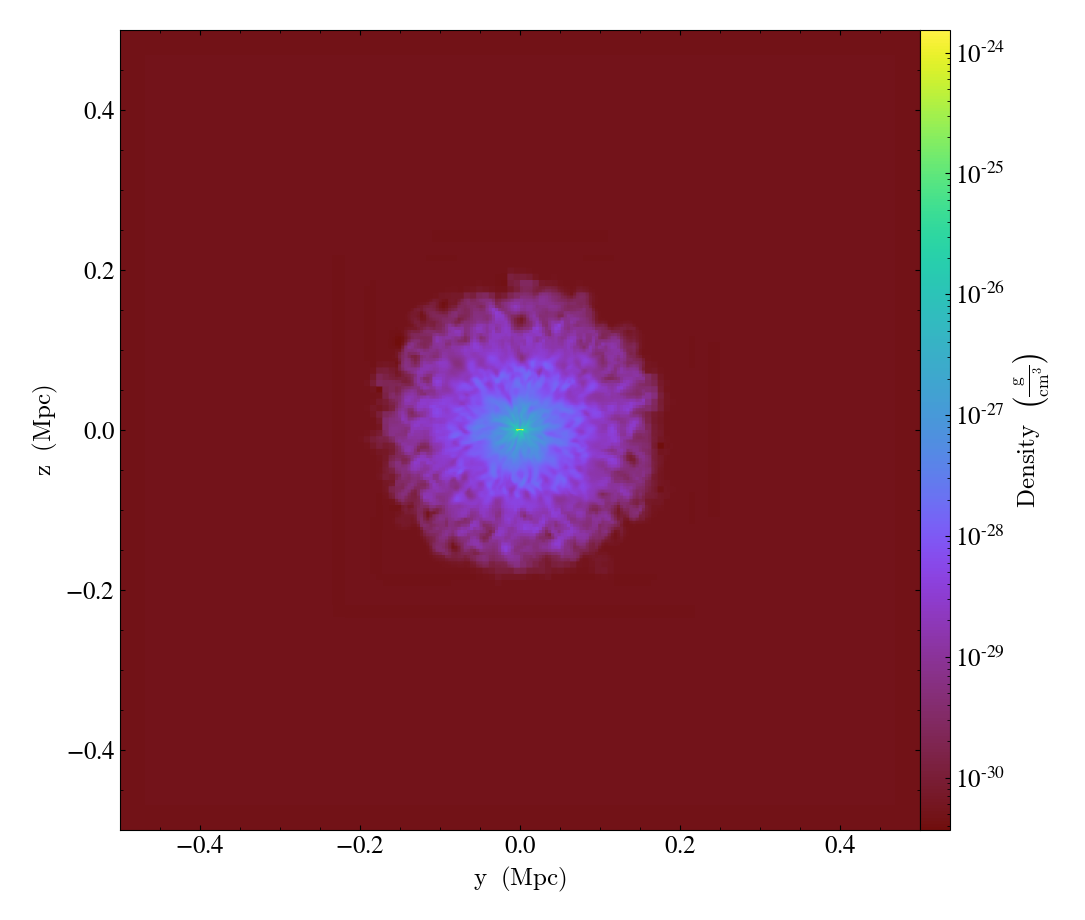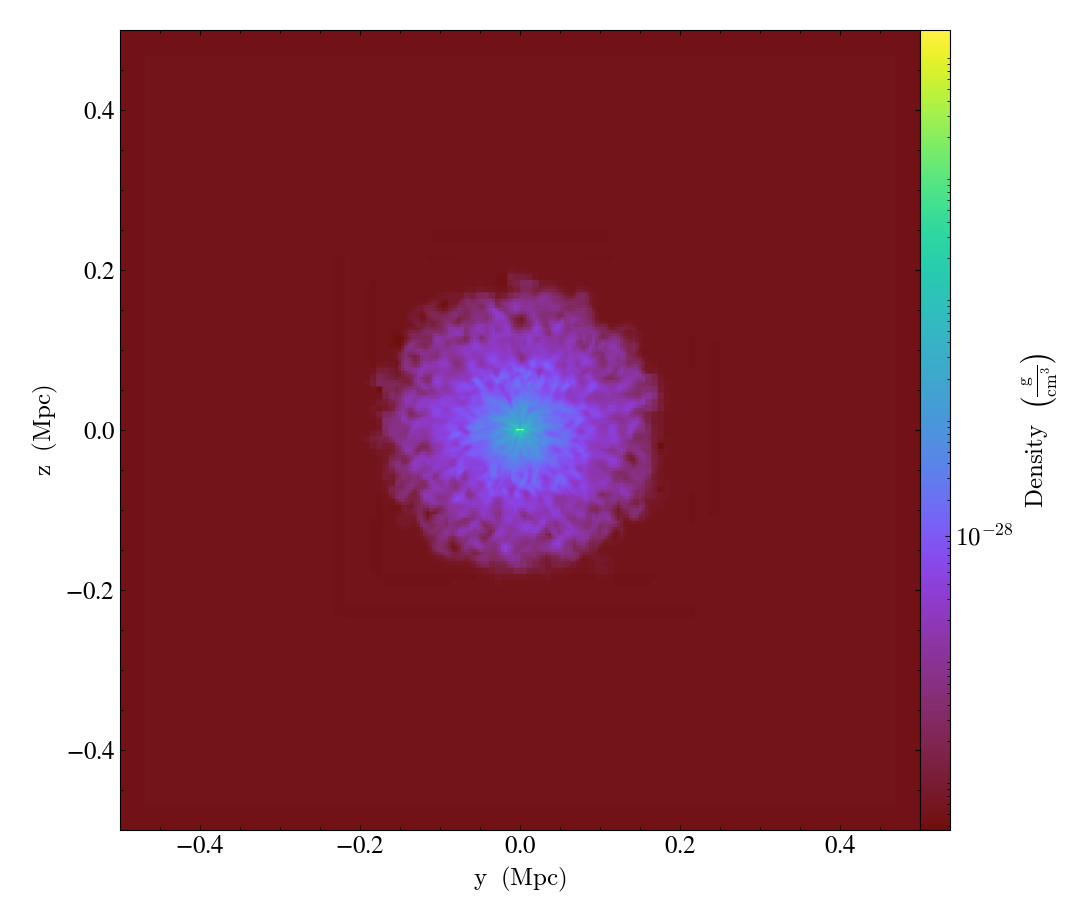# Custom Colorbar Tickmarks¶

Notebook
In :
import yt

In :
ds = yt.load("IsolatedGalaxy/galaxy0030/galaxy0030")
slc = yt.SlicePlot(ds, "x", ("gas", "density"))
slc

Out:PlotWindow plots are containers for plots, keyed to field names. Below, we get a copy of the plot for the Density field.

In :
plot = slc.plots[("gas", "density")]


The plot has a few attributes that point to underlying matplotlib plot primitives. For example, the colorbar object corresponds to the cb attribute of the plot.

In :
colorbar = plot.cb


Next, we call _setup_plots() to ensure the plot is properly initialized. Without this, the custom tickmarks we are adding will be ignored.

In :
slc._setup_plots()


To set custom tickmarks, simply call the matplotlib set_ticks and set_ticklabels functions.

In :
colorbar.set_ticks([1e-28])
colorbar.set_ticklabels(["$10^{-28}$"])
slc

Out: